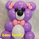66352 views
Published just for Goldminds members .
Open-source script

In true TradingView spirit, the author of this script has published it open-source, so traders can understand and verify it. Cheers to the author! You may use it for free, but reuse of this code in a publication is governed by House Rules. You can favorite it to use it on a chart.

Want to use this script on a chart?
```//
// @author LazyBear
// If you use this code in its orignal/modified form, do drop me a note.
//
// Modified by Bromley

study("TDI - Traders Dynamic Index [Goldminds]", shorttitle="TDIGM")

rsiPeriod = input(11, minval = 1, title = "RSI Period")
bandLength = input(31, minval = 1, title = "Band Length")
lengthrsipl = input(1, minval = 0, title = "Fast MA on RSI")
lengthtradesl = input(9, minval = 1, title = "Slow MA on RSI")

src = close                                                             // Source of Calculations (Close of Bar)
r = rsi(src, rsiPeriod)                                                 // RSI of Close
ma = sma(r, bandLength)                                                 // Moving Average of RSI [current]
offs = (1.6185 * stdev(r, bandLength))                                  // Offset
up = ma + offs                                                          // Upper Bands
dn = ma - offs                                                          // Lower Bands
mid = (up + dn) / 2                                                     // Average of Upper and Lower Bands
fastMA = sma(r, lengthrsipl)                                            // Moving Average of RSI 2 bars back
slowMA = sma(r, lengthtradesl)                                          // Moving Average of RSI 7 bars back

hline(30)                                                               // Oversold
hline(50)                                                               // Midline
hline(70)                                                               // Overbought

upl = plot(up, "Upper Band", color = blue)                              // Upper Band
dnl = plot(dn, "Lower Band", color = blue)                              // Lower Band
midl = plot(mid, "Middle of Bands", color = orange, linewidth = 2)      // Middle of Bands

plot(slowMA, "Slow MA", color=green, linewidth=2)                       // Plot Slow MA
plot(fastMA, "Fast MA", color=red, linewidth=2)                         // Plot Fast MA

fill(upl, midl, red, transp=90)                                         // Fill Upper Half Red
fill(midl, dnl, green, transp=90)                                       // Fill Lower Half Green```